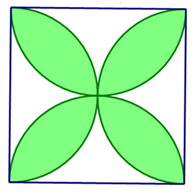# Circles and Square

Directions: The diagram shows a square and four semicircles formed using each side of the square as a diameter. What fraction of the square is shaded?### Hint

How can you partition the space to find the area you need?

(Π-2)/2  (Thanks to Tracy Parry for correcting the answer)

Source: Dylan Kane

## Two Step Inequality with Fractional Coeffcient

Directions: Using the digits 1 to 7 at most one time each, place a digit …

1.I’m stumped, can someone shed some light on where to start and where to partition?

•Divide it into fourths and find the space between the smaller square and the quarter of a circle. Once partitioned you can see that there are eight of these empty spaces which comprise the non-shaded space.

2.My grade 8 students and I still don’t fully understand the answer that is given. Is there anyone who might be able to show us how they got to this answer?

Thank you!!

3.If we are getting the fraction, the four shaded areas / the square, I am pretty sure the answer is (pi-2)/4. (denominator is not 2).

•Sorry! I got it. (Π-2)/2 is right!